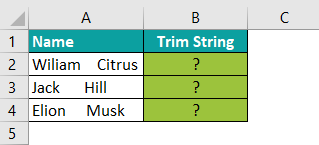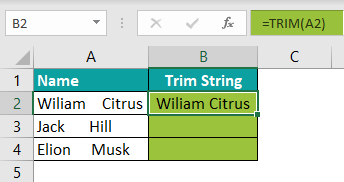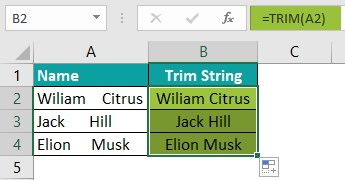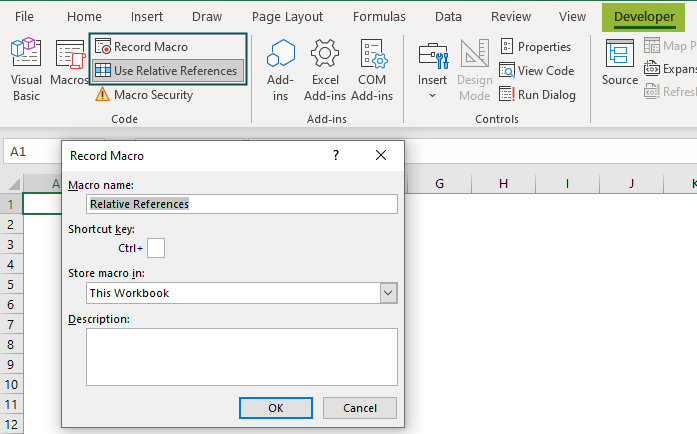# Relative References in ExcelArticle byExcelMojo Team## What Is Relative References In Excel?

The Relative References in Excel enable users to copy the same formula and perform calculations easily as it automatically changes the values concerning the row or column. The formula can be copied manually or with the AutoFill option in Excel. It is used whenever a formula has to be repeated.

For example, the following table has values in column A. Here, we will try to multiply the value in cell A2 by 10 by using it as a relative reference.

Enter the formula =A2*10 in cell B2 to get the result of 10.

If we copy the formula entered in cell B2 in cell B3, “=A2*10” gets adjusted as =A3*10. Thus, A2 acts as a relative cell reference in this example.

###### Key Takeaways
• The relative references in Excel are referencing tools that change the cell references when the formula is copied to another cell.
• Relative references automatically substitute the value based on the cell where the formula is copied.
• It is employed whenever you need to repeat a formula, such as SUM, TRIM, etc.
• The relative references in Excel can be used with two other cell reference types – absolute and mixed.

### How To Use Relative References In Excel?

Here is a basic example to understand how to use relative references in excel.

Consider the below table that shows the numbers (in columns A and B). Next, the sum of these numbers has to be calculated. Let us understand the mechanism of the relative references in Excel with the following steps:

Step 1:  Select an empty column (column C) for displaying the output.

Step 2:  Enter the SUM formula in cell C2

=SUM(A2,B2)

Type the SUM function =SUM followed by the opening parenthesis to enter this formula. Next, select the cells A2 & B2 in the SUM excel formula.

Step 3:  Press the ‘Enter’ key. The output in cell C2 is returned as 30, as shown in the following image. So, the sum of the numbers (in A2 and B2) is calculated as 30.

Step 4: We have to apply the same formula in a row “3”. Cells A3 and B3 have two numbers, and we need to calculate the sum in cell C3.

Step 5: We can achieve this in two ways: write the SUM formula in cell C3 as =SUM(A3, B3) and get the result. Or, copy the formula from cell C2 and paste it into cell C3.

Step 6: When we copy and paste, the formula is only copied, not that cell’s value.

• Hence, when the formula is copied from cell C2 to cell C3, the formula automatically changes in the selected cell. Thus, it is a relative cell reference in Excel.

### Examples

Let us look at some advanced examples of relative references in excel to understand its working:

#### Example #1 – Relative References in Excel

The below image shows the list of fruits (in column A) and their Price/Unit (in column B) and Unit details (in column C). First, we need to calculate the total cost of fruits. Let us understand how the relative references in Excel work in the following steps.

Step 1: Select a blank column (column D)  to get the output.

Step 2:  Enter the formula =B2*C2 in cell D2

We can also type “=” and select cells B2 & C2 to enter the cell references in the formula.

Step 3:  Press the “Enter” key. The output in cell D2 is \$10,000, as shown in the following image.

So, the total cost per price/unit and unit of lemon is \$10,000.

Step 4: We have to calculate the total cost of other fruits in cell range A3:A6 using the same formula. For example, in row 3, cells B3 and C3 have the values as Price/unit and Unit, and we need to calculate the total cost of the banana in cell D3.

Step 5: We can achieve this in two different ways:

1. We can write the formula in cell D3 as =B3*C3 and obtain the result.
2. Or we can copy cell D2 and paste it into cell D3.

Step 6: When we copy and paste the formula from D2, only the formula gets copied. The cell value gets updated with the values in the respective row.

Step 7: Press Enter key. Drag the formula downwards using the autofill option till cell D6, as shown in column E in the following image.

• The total cost of various fruits is obtained by simply copying the formula from cell D2 to cell D6. The cell references in the formula get substituted with the respective values. It is relative cell references in Excel.

#### Example #2 – With Absolute References

Each cell has a unique cell reference in excel. These are known as cell locations. An absolute reference is a referencing tool that locks a cell reference in the formula such that the cell location remains the same even when the formula is copied. On the other hand, the relative cell references automatically substitute the cell references when a formula is copied.

The absolute reference is used when the formula has a dollar sign (“\$”).

There are three different absolute references in excel:

• Absolute Reference for column and row
• For example is “\$A\$1,” where both column and row are locked.
• Absolute Reference for only column
• For example, “\$A1.”
• Here,  only the column is locked.
• Absolute Reference for only row
• For example, “A\$1,” where only the row remains locked.

The following image shows the list of items (in column A), their prices (in column B), and the deductible tax of \$50 in cell C7. Next, we need to calculate the total price of each item (in column D). We use the following steps in Excel to understand the Absolute References in Excel.

Step 1: We have to select a blank column for displaying the output, such as column C.

Step 2:  Enter the formula in cell C2:

=B2-\$C\$7

We can also type “=,” and then select the cells B2 and C7 to enter the cell references in the formula. For absolute reference, we must insert the “\$” dollar sign as \$C\$7.

Step 3:  Press the “Enter” key. The output of \$950 is obtained in cell C2, as shown in the following image.

So, the total price of cake in cell A2 after subtracting the deductible tax is calculated.

Step 4: We have to repeat the same procedure in row 3. Cell B3 and locked cell \$C\$7 have the Price and Deductible Tax values, and we need to calculate the total price using the data in cell C3.

Step 5: We can achieve this in two different ways: write the formula in cell C3 as =B3-\$C\$7 and get the result or copy the formula from cell C2 and paste it to cell C3.

Step 6: The cells’ value is not copied when we copy and paste. Instead, only the formula gets copied.

Step 7: Press the “Enter” key. Then, drag the formula downwards using the autofill option till cell C6, as shown in column D in the following image.

• Hence, the cell location does not change after putting the “\$” dollar sign in the formula =B2-\$C\$7. However, the cell address of column B has changed automatically as the formula is copied from cell C2 to cell C6.

Thus, we can understand how Excel’s relative and absolute cell References work.

#### Example #3 – With Mixed References

The mixed references in Excel are used for the column or the row. It only refers to the column or row references. Both the relative and absolute references are used in mixed references in Excel.

Consider the below table with values in columns A and B. First, let us calculate the total value. This example helps us understand how mixed references in Excel function.

Step 1: We have to select blank cells to display the output. Let us choose cells C2 and D2.

Step 2:  Enter the SUM formula in cell C2.

=SUM(\$A:A)

Next, we will add \$A:A as the cell references in the SUM formula.

Step 3:  Press the “Enter” key. We get the output in cell C2, as shown in the following image.

So, the total is obtained as 60.

Step 4: We will use the same formula in column D.

Column A is an absolute reference as \$A, but “A” is a relative reference. So, the values are added, but the value of \$A does not change. So the value remains at 60. To calculate the total, “A” changes into “B.” So the values in cell D2 become 60+10+20+30=120.

We can copy cell C2 and paste it to cell D2.

Step 5: When we copy and paste the formula, the cell’s value is not copied. Only the formula gets copied, as shown in the following image.

• Hence, the cell location in the formula =SUM(\$A:B) does not change after inserting the “\$” dollar sign.

But, the cell address of column A has changed automatically when the formula is copied from cell C2 to cell D2.

Therefore, this example proves that the mixed references in excel use both relative and absolute cell references in Excel.

### Important Things To Note

• Every cell in excel is a relative reference cell.
• The cell references in the cell address and formula change automatically when the formula is copied in another cell.

What does using relative references mean in Excel?

The relative references in Excel represent the location of the cell. Therefore, it allows users to effortlessly replicate the same formula and do calculations because the numbers are automatically changed concerning the row or column.

How to use Relative References in Excel?

Let us consider an example to understand how to use the relative references in Excel. Column A contains Names with extra spaces.

The steps for using this function are listed as follows:Trim the text strings using the TRIM formula in cell B2.The formula is copied from cell B2 to cells B3 to B4.

The formula =TRIM(A2) gets adjusted with relative references in Excel. The formula in cell B3 changes to =TRIM(A3), and in cell B4, the formula changes to =TRIM(A4).How to turn on relative references in Excel?

a) Click ‘Use Relative References’ from the Developer tab of Excel.
b) Choose the ‘Record Macro’ option.
c) Specify the Macro name as relative references in the ‘Record Macro’ window.
d) Click ‘OK’ to start recording the relative reference macro.